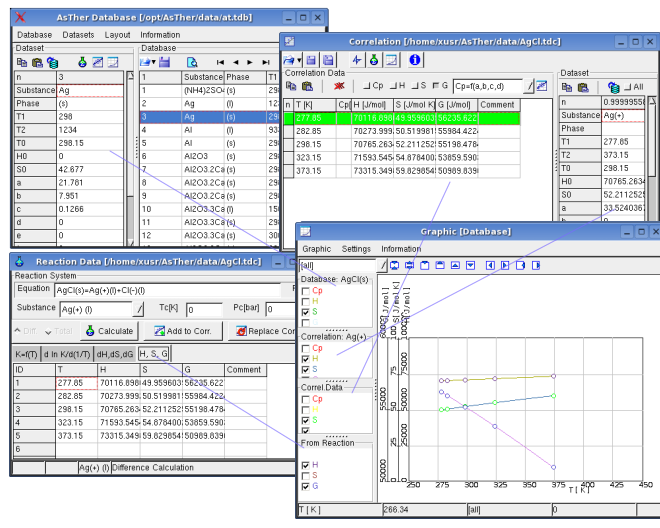Content
1.1. Database-Window definition of a new dataset
1.2. Correlation-Window  creation datasets using the values of the state function
1.3. Reaction-Data-Window: calculation  the values of the state function using equilibrium data of reactions
1.4. Graphic-Window display the datasets in Database, Correlation and Reaction  Windows

The application allows to create and to change thermodynamic datasets

1.1. Database-Window

1.1.1. General Information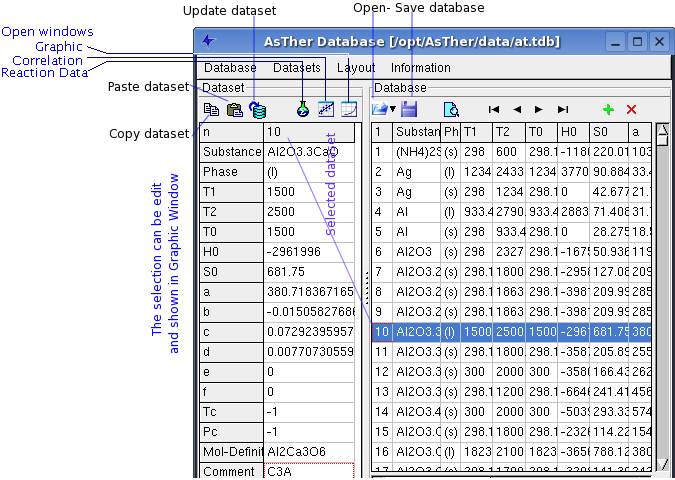Database -> New: creates a new data source
Database -> Open: opens an existing database
Database -> Add: adds an another database to the current database. No check for redundancy of datasets will be made!
Database -> Close: closes the active database file.

Dataset -> Select by double click:
When it is checked,  the data sets can be selected only by mouse double click or by enter-key on keyboard.
When it is not checked, the data sets can be selected by simple mouse click or arrow-keys on keyboard
You can select data sets by navigations buttons.

Dataset -> Search: opens a dialog box to search the dataset for given criteria
When the checkbox exact is not activated, data items of string format will be listed according to next match of the respective character sequence.
The temperature range relates to the validity of the data.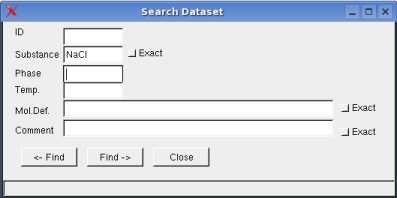Dataset -> Syntax Check: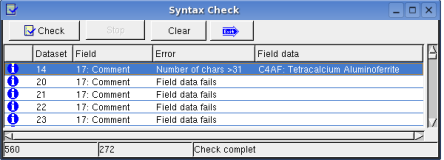Dataset -> Sort:  sorts dataset alphabetic.
Dataset -> Renew Index: The ID of the datasets  will be changed in ascending order

Information
Information -> Messages: shows the Message Window
Information -> Help: shows this text in browser, which is given in AsTher.sys

1.2. Correlation-Window

1.2.1. General InformationA new thermodynamic dataset can be created using single data of state functions,
when Cp-, H-, S-, or G- values at different temperatures are known.
Enter the known data into the respective columns.
If datasets for gases are to create according to real gas rules, "(g)" must be written in the field "Phase" and Tc and Pc must be known.

The correlation can be calculated using Cp, H and/or G values.
The corresponding Check Boxes must be checked (active).
The temperature must always known.
One or more values of Cp, H, S, G must be given in the Correlation-Dataset.
When H, S or G are included, the reference values T0, H0, S0  must be given.

The Cp Function is defined by:
Cp= a + b . 0.001 . T + c .  106 . T -2  + d . 10 -6 . T2 + e .  109 . T -3 + f . 10 -9 . T3
or
Cp= a + b . (T/1000) + c .   (T/1000) -2  + d . (T/1000)2 + e .  (T/1000) -3 + f . (T/1000)3

According to the selection in the example above, the correlation will calculate only the coefficients a, b, c, and d . These selections should be changed until a sufficiently high correlations-coefficient is reached. This coefficient ranges from 0 to 10 : no relation; 1: best correlation . A comparison of given and calculated values in the valid temperature range should serve as double check.
Many data sources list values for enthalpy, entropy and free energy according to the law of ideal gas. If these data are taken, only the Cp-values should be correlated in order to create a complete dataset for real gas behaviour.

In AsTher, the standard state is defined at 1 bar and 298.15 K, i.e.: enthalpy of a pure substance at this state is zero.
If data related to a different standard definition are taken for the correlation the results will not be compatible with datasets of AsTher.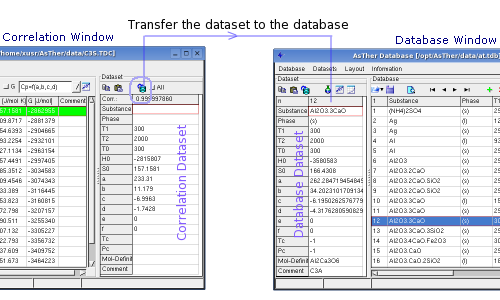You can transfer the Correlation-Dataset  to the Database-Dataset.
When "All" checked, the data will be replaced in the table Database-Dataset, even if a fields in the Correlation-Dataset does not contain any entry.
When "All" not checked, the fields will not replaced data in the Database-Dataset, when the corresponding filed in Correlation-Dataset contains no any data (blank).

1.3. Reaction-Data-Window  - Calculation of the State Functions

1.3.1. General Information
If the equilibrium constant of a reaction is known, the H, S and G values of a substance can be determined corresponding to the Van' t Hoff – relationsWhen the state function calculated in this window, the data can be transferred to the correlation-window and the dataset can be created.
In this window, the equilibrium constant will be linearly correlated vs. temperature on the basis of the entered data on page K(T). The correlation can be conducted either totally or differentially. In case of smaller temperature ranges, the correlation should be carried out totally. With wider temperature ranges, a total correlation can produce erroneous results. As results from the correlation K and ΔH will be calculated and displayed on page K(T)Int. Based on those values for K and H, S and G are calculated and displayed on page dH,dS,dG and likewise H, S, G on page H,S,G. If the differential values for dH,dS,dG are known for a reaction, those can be entered on the respective page. In this case, the inputs on page Kf(T) will not be considered and there will be no value displayed on page K(T)Int. If the calculation is conducted successfully, the data for H, S, G can be transferred to the correlation table together with the temperature values. Press button replace to replace the data in the correlation table by those values. If the dialog box is closed by Button add, the calculated values will be added to the correlation table without replacing the existing data.

1.3.2. Example

In following, the data are entered in the Sheet Kf(T)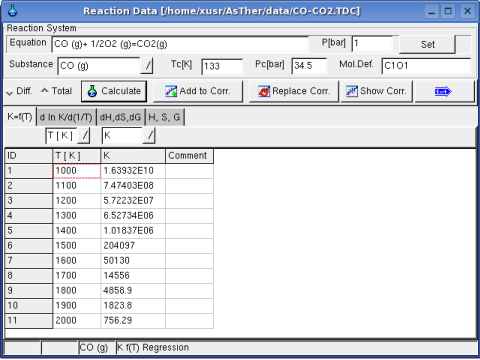Regression option is for differential or total correlation selected, and the button Calculate pressed.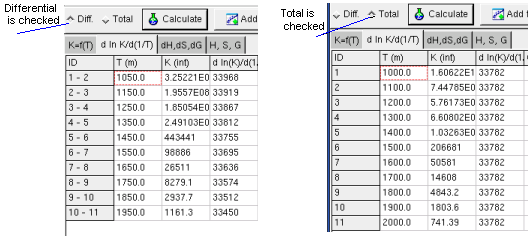The Sheet dH, dS, dG shows calculated change of the state functions by reaction, when the K and T values given in the first sheet (Kf(T)).

When K and T values are unknown, but the several values of dH and/or dS and/or dG are known,
these values can be are entered in the  the Sheet dH, dS, dG.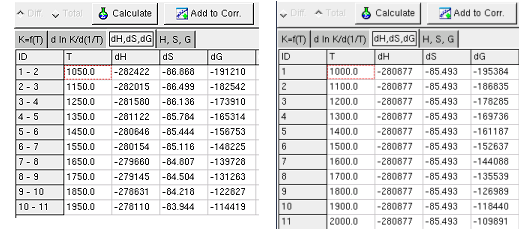When the button calculate is pressed and the Sheet dH, dS, dG  is shown, the calculation carried out only with the data in this sheet. The values of the state function are shown in the Sheet H, S, G .

In the Sheet dH, dS, dG, not all values of ΔH, ΔS and ΔG of a reaction have to be entered.
When ΔH, ΔS entered without ΔG , the application calculates ΔG corresponding  ΔG = ΔH-ΔS.
When ΔS, ΔG entered without ΔH , the application calculates ΔH corresponding  ΔG = ΔH-ΔS.

When only one of ΔH or ΔS or ΔG is entered, the application calculate only corresponding  H or S or G,1.4.  Graphic-Window

1.4.1. General Information